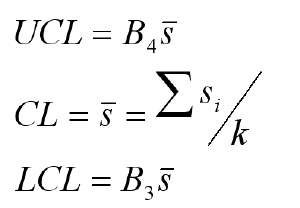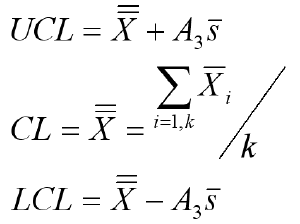# XbarS Chart Formulas

The XbarS Chart can help you evaluate the stability of processes using variable data--time, cost, length, weight when you have 6 or more samples per period.

### XbarS Chart formulas used in QI Macros SPC add-in for Excel

XbarS Standard Deviation Chart FormulaAverage (Xbar) Chart Formulak = number of subgroups (a group of measurements produced under the same set of conditions)

n = number of samples in a subgroup

A3, B3 and B4 are constants based on n

Conforms with ANSI/ASQC B1, B2, B3 1996

Process: Calculate, plot, and evaluate the Range Chart first. If it is "out of control," so is the process. If the Range Chart looks okay, then calculate, plot, and evaluate the X Chart.

Note: Some people wonder why QI Macros results are a tiny bit different from some versions other software. The answer is that they use a different estimator. Donald Wheeler in Advanced Topics in Statistical Process Control says: "Even though the Pooled [Standard Deviation] estimator is common in many applications, the robustness of Rbar/d2 and Sbar/c4 makes them the preferred estimators for use with process behavior charts."

Furthermore, Minitab started using Pooled Standard Deviation to calculate Cp/Cpk, and control limits on XbarR and XbarS Charts in versions 15 and 16. Minitab 17 went back to Rbar/d2 and Sbar/c4 for XbarR/S control limits, but retained pooled stdev for Cp/Cpk calculations when using multiple samples.

The XbarS Chart is just one of the tools included in the QI Macros for Excel SPC Software for Excel.

### Why Choose QI Macros Over Other Control Chart Software?### Affordable

• Only \$329 USD - less with quantity discounts
• No annual subscription fees
• Free technical support### Easy to Use

• Works right in Excel
• Create a chart in seconds
• Easy to customize & share charts### Proven and Trusted

• More than 100,000 users
• In More than 80 countries
• Five Star CNET Rating - Virus free